# CTC算法概念

CTC算法全称叫：Connectionist temporal classification。从字面上理解它是用来解决时序类数据的分类问题。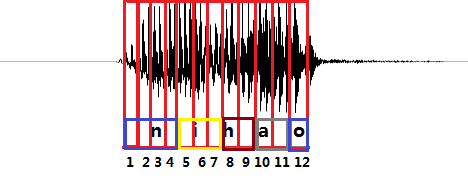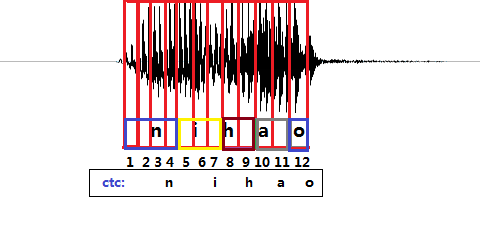CTC引入了blank（该帧没有预测值），每个预测的分类对应的一整段语音中的一个spike（尖峰），其他不是尖峰的位置认为是blank。对于一段语音，CTC最后的输出是spike（尖峰）的序列，并不关心每一个音素持续了多长时间。

# RNN+CTC模型的训练

$z=\left({z}_{1},{z}_{2},{z}_{3},...{z}_{U}\right)$$z=(z_1,z_2, z_3,...z_U)$表示这段样本语音对应的正确的音素。比如，一段发音“你好”的声音，经过MFCC计算后，得到特征$x$$x$， 它的文本信息是“你好”，对应的音素信息是$z=\left[n,i,h,a,o\right]$$z=[n,i,h,a,o]$(这里暂且将每个拼音的字母当做一个音素)。

$\sum _{t-1}^{T}{y}_{k}^{t}=1,{y}_{k}^{t}\ge 0$$\sum_{t-1}^{T}y^t_k=1, y^t_k\geq0$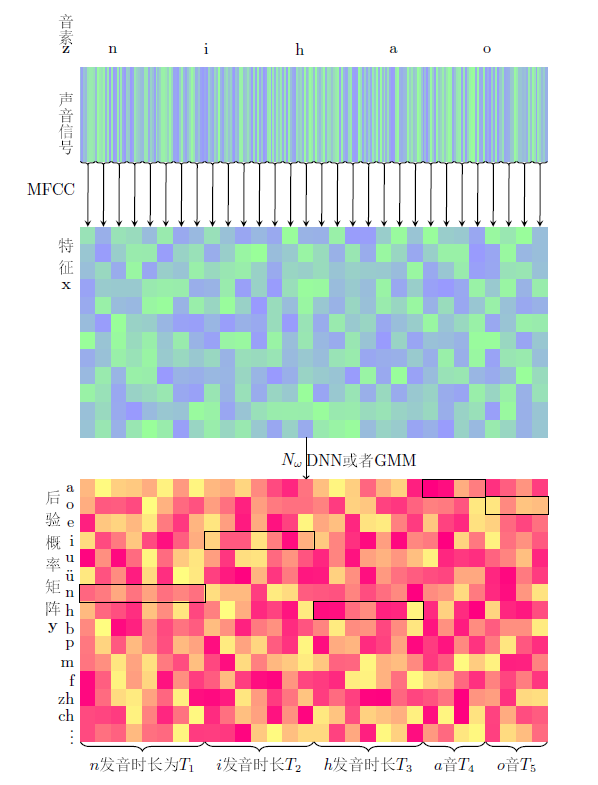## 路径π$\pi$$\pi$和B变换

$z\mathrm{\prime }=\left[\underset{{T}_{1}}{\underset{⏟}{n,n,n,...,n}},\underset{{T}_{2}}{\underset{⏟}{i,i,i,...i}},\underset{{T}_{3}}{\underset{⏟}{h,h,h,...h}},\underset{{T}_{4}}{\underset{⏟}{a,a,a,...,a}},\underset{{T}_{5}}{\underset{⏟}{o,o,o,...,o}}\right]$$z\prime = [\underbrace{n,n,n,...,n}_{T_1},\underbrace{i,i,i,...i}_{T_2},\underbrace{h,h,h,...h}_{T_3},\underbrace{a,a,a,...,a}_{T_4},\underbrace{o,o,o,...,o}_{T_5}]$

${T}_{1}+{T}_{2}+{T}_{3}+{T}_{4}+{T}_{5}=T$$T_1+T_2+T_3+T_4+T_5 = T$

$p\left(z\mathrm{\prime }|x\right)=p\left(z\mathrm{\prime }|y={N}_{w}\left(x\right)\right)={y}_{z{\mathrm{\prime }}_{1}}^{1}{y}_{z{\mathrm{\prime }}_{2}}^{2}{y}_{z{\mathrm{\prime }}_{3}}^{3}....{y}_{z{\mathrm{\prime }}_{T}}^{T}$$p(z\prime|x) = p(z\prime| y = N_w(x)) = y^1_{z\prime_1}y^2_{z\prime_2}y^3_{z\prime_3}....y^T_{z\prime_T}$ $\phantom{\rule{2em}{0ex}}\phantom{\rule{2em}{0ex}}\phantom{\rule{2em}{0ex}}\phantom{\rule{2em}{0ex}}\phantom{\rule{2em}{0ex}}\phantom{\rule{2em}{0ex}}\phantom{\rule{2em}{0ex}}\phantom{\rule{2em}{0ex}}\phantom{\rule{2em}{0ex}}\phantom{\rule{2em}{0ex}}$$\qquad\qquad\qquad\qquad\qquad\qquad\qquad\qquad\qquad\qquad$ (1)

$mi{n}_{w}-log\left({y}_{{z}_{1}^{\mathrm{\prime }}}^{1}.{y}_{{z}_{2}^{\mathrm{\prime }}}^{2}.{y}_{{z}_{3}^{\mathrm{\prime }}}^{3}...{y}_{{z}_{T}^{\mathrm{\prime }}}^{T}\right)$$min_w -log(y^1_{z^\prime_1}.y^2_{z^\prime_2}.y^3_{z^\prime_3}...y^T_{z^\prime_T})$ $\phantom{\rule{2em}{0ex}}\phantom{\rule{2em}{0ex}}\phantom{\rule{2em}{0ex}}\phantom{\rule{2em}{0ex}}\phantom{\rule{2em}{0ex}}\phantom{\rule{2em}{0ex}}\phantom{\rule{2em}{0ex}}\phantom{\rule{2em}{0ex}}\phantom{\rule{2em}{0ex}}\phantom{\rule{2em}{0ex}}$$\qquad\qquad\qquad\qquad\qquad\qquad\qquad\qquad\qquad\qquad$ (2)

subject to: $y={N}_{w}\left(x\right)$$y = N_w(x)$$\phantom{\rule{2em}{0ex}}\phantom{\rule{2em}{0ex}}\phantom{\rule{2em}{0ex}}\phantom{\rule{2em}{0ex}}\phantom{\rule{2em}{0ex}}\phantom{\rule{2em}{0ex}}\phantom{\rule{2em}{0ex}}\phantom{\rule{2em}{0ex}}\phantom{\rule{2em}{0ex}}\phantom{\rule{2em}{0ex}}$$\qquad\qquad\qquad\qquad\qquad\qquad\qquad\qquad\qquad\qquad$ (3)

$\frac{\mathrm{\partial }-log\left({y}_{{z}_{1}^{\mathrm{\prime }}}^{1}.{y}_{{z}_{2}^{\mathrm{\prime }}}^{2}.{y}_{{z}_{3}^{\mathrm{\prime }}}^{3}...{y}_{{z}_{T}^{\mathrm{\prime }}}^{T}\right)}{\mathrm{\partial }{y}_{k}^{t}}=$$\frac{\partial - log(y^1_{z^\prime_1}.y^2_{z^\prime_2}.y^3_{z^\prime_3}...y^T_{z^\prime_T})}{\partial y^t_k} =$$\left\{\begin{array}{l}-\frac{{y}_{z{\mathrm{\prime }}_{1}}^{1}...{y}_{z{\mathrm{\prime }}_{i-1}}^{i-1}.{y}_{z{\mathrm{\prime }}_{i+1}}^{i+1}....{y}_{z{\mathrm{\prime }}_{T}}^{T}}{{y}_{{z}_{1}^{\mathrm{\prime }}}^{1}.{y}_{{z}_{2}^{\mathrm{\prime }}}^{2}.{y}_{{z}_{3}^{\mathrm{\prime }}}^{3}...{y}_{{z}_{T}^{\mathrm{\prime }}}^{T}},\phantom{\rule{2em}{0ex}}if\phantom{\rule{2em}{0ex}}k=z{\mathrm{\prime }}_{i}\phantom{\rule{2em}{0ex}}and\phantom{\rule{2em}{0ex}}t=i\\ 0\phantom{\rule{2em}{0ex}}其他\end{array}$$\begin{cases} -\frac{y^1_{z\prime_1}...y^{i-1}_{z\prime_{i-1}}.y^{i+1}_{z\prime_{i+1}}....y^T_{z\prime_T}}{y^1_{z^\prime_1}.y^2_{z^\prime_2}.y^3_{z^\prime_3}...y^T_{z^\prime_T}} , \qquad if \qquad k = z\prime_i \qquad and \qquad t=i \\ 0 \qquad 其他\end{cases}$

$L=\left\{a,o,e,i,u,\stackrel{ˇ}{u},b,p,m,...\right\}$$L=\lbrace a, o, e, i, u, \check{u},b,p,m,...\rbrace$

$\pi =\left({\pi }_{1},{\pi }_{2},{\pi }_{3},...,{\pi }_{T}\right),{\pi }_{i}\in L$$\pi= (\pi_1, \pi_2, \pi_3, ..., \pi_T), \pi_i \in L$

${\pi }^{1}=\left(j,j,i,n,y,y,e,e,w,w,u,u,u,r,r,e,e,n,n,r,r,u,u,sh,sh,u,u,i,i\right)$$\pi^1= (j,j,i,n,y,y,e,e,w,w,u,u,u,r,r,e,e,n,n,r,r,u,u,sh,sh,u,u,i,i)$
${\pi }^{2}=\left(n,n,n,n,i,i,i,i,h,h,h,h,a,a,a,a,a,a,a,a,a,o,o,o,o,o,o,o,o,o\right)$$\pi^2= (n,n,n,n,i,i,i,i,h,h,h,h,a,a,a,a,a,a,a,a,a,o,o,o,o,o,o,o,o,o)$
${\pi }^{3}=\left(h,h,h,h,h,h,a,a,a,a,a,a,a,o,o,o,o,n,n,n,n,n,n,i,i,i,i,i,i,i\right)$$\pi^3= (h,h,h,h,h,h,a,a,a,a,a,a,a,o,o,o,o,n,n,n,n,n,n,i,i,i,i,i,i,i)$
${\pi }^{4}=\left(n,i,h,a,o,o,o,o,o,o,o,o,o,o,o,o,o,o,o,o,o,o,o,o,o,o,o,o,o,o,o\right)$$\pi^4= (n,i,h,a,o,o,o,o,o,o,o,o,o,o,o,o,o,o,o,o,o,o,o,o,o,o,o,o,o,o,o)$
${\pi }^{5}=\left(n,n,n,n,n,n,n,n,n,n,n,n,n,n,n,n,n,n,n,n,n,n,n,n,i,h,a,o\right)$$\pi^5= (n,n,n,n,n,n,n,n,n,n,n,n,n,n,n,n,n,n,n,n,n,n,n,n,i,h,a,o)$
${\pi }^{6}=\left(n,n,n,i,i,i,h,h,h,h,h,a,,o,o,o,o,o,o,o,o,o,o,o,o,o,o,o,o,o,o\right)$$\pi^6= (n,n,n,i,i,i,h,h,h,h,h,a,,o,o,o,o,o,o,o,o,o,o,o,o,o,o,o,o,o,o)$

$B\left(a,a,a,b,b,b,c,c,d\right)=\left(a,b,c,d\right)$$B(a,a,a,b,b,b,c,c,d) = (a,b,c,d)$

$B\left({\pi }^{1}\right)=\left(j,i,n,y,e,w,u,r,e,n,r,u,s,h,u,i\right)$$B(\pi^1) = (j,i,n,y,e,w,u,r,e,n,r,u,s,h,u,i)$
$B\left({\pi }^{2}\right)=\left(n,i,h,a,\right)$$B(\pi^2) = (n,i,h,a,)$
$B\left({\pi }^{3}\right)=\left(h,a,o,n,i\right)$$B(\pi^3) = (h,a,o,n,i)$
$B\left({\pi }^{4}\right)=\left(n,i,h,a,o\right)$$B(\pi^4) = (n,i,h,a,o)$
$B\left({\pi }^{5}\right)=\left(n,i,h,a,o\right)$$B(\pi^5) = (n,i,h,a,o)$
$B\left({\pi }^{6}\right)=\left(n,i,h,a,o\right)$$B(\pi^6) = (n,i,h,a,o)$

$p\left(\pi |x\right)=p\left(\pi |y={N}_{w}\left(x\right)\right)=p\left(\pi |y\right)=\prod _{t=1}^{T}{y}_{{\pi }_{t}}^{t}$$p(\pi|x) = p(\pi| y = N_w(x)) = p(\pi| y)=\prod_{t=1}^{T} y^t_{\pi_t}$

$ma{x}_{w}p\left(z|y={N}_{w}\left(x\right)\right)=p\left(z|x\right)=\sum _{B\left(\pi \right)=z}p\left(\pi |x\right)$$max_w p(z|y=N_w(x)) = p(z|x)= \sum_{B(\pi)=z}p(\pi|x)$ $\phantom{\rule{2em}{0ex}}\phantom{\rule{2em}{0ex}}\phantom{\rule{2em}{0ex}}$$\qquad\qquad\qquad$ (4)

## 训练实施方法

CTC的训练过程是通过$\frac{\mathrm{\partial }p\left(z|x\right)}{\mathrm{\partial }w}$$\frac {\partial p(z|x)}{\partial w}$调整w的值使得4中的目标值最大，而计算的过程如下：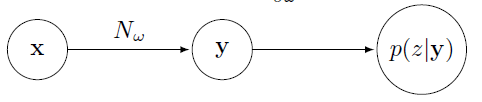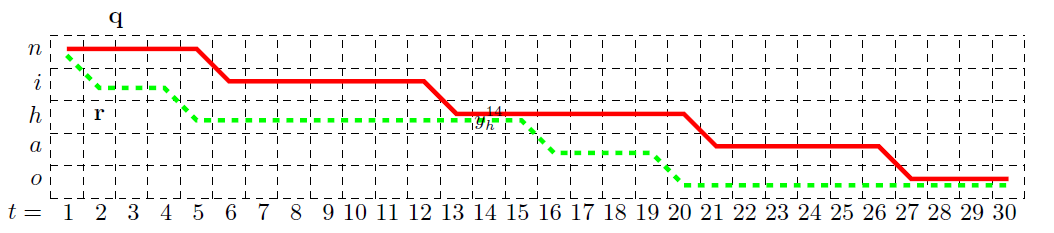$\frac{\mathrm{\partial }p\left(z|y\right)}{\mathrm{\partial }{y}_{h}^{14}}$$\frac {\partial p(z|y)}{\partial y^{14}_h}$

= $\frac{\mathrm{\partial }\sum _{B\left(\pi \right)=z}p\left(\pi |y\right)}{\mathrm{\partial }{y}_{h}^{14}}$$\frac {\partial \sum_{B(\pi)=z}p(\pi|y)}{\partial y^{14}_h}$

= $\frac{\mathrm{\partial }\sum _{B\left(\pi \right)=z}\prod _{t=1}^{T}{y}_{{\pi }_{t}}^{t}}{\mathrm{\partial }{y}_{h}^{14}}$$\frac {\partial \sum_{B(\pi)=z}\prod_{t=1}^T y^t_{\pi_t}}{\partial y^{14}_h}$
=$\frac{\stackrel{和{y}_{h}^{14}有关的项}{\stackrel{⏞}{\mathrm{\partial }\sum _{B\left(\pi \right)=z,{\pi }_{14}=h}\prod _{t=1}^{T}{y}_{{\pi }_{t}}^{t}}}+\stackrel{和{y}_{h}^{14}无关的项}{\stackrel{⏞}{\mathrm{\partial }\sum _{B\left(\pi \right)=z,{\pi }_{14}\ne h}\prod _{t=1}^{T}{y}_{{\pi }_{t}}^{t}}}}{\mathrm{\partial }{y}_{h}^{14}}$$\frac {\overbrace{\partial \sum_{B(\pi)=z,\pi_{14}=h}\prod_{t=1}^T y^t_{\pi_t}}^{和y^{14}_h有关的项} + \overbrace{\partial \sum_{B(\pi)=z,\pi_{14} \neq h}\prod_{t=1}^T y^t_{\pi_t}}^{和y^{14}_h无关的项}}{\partial y^{14}_h}$

=$\frac{\mathrm{\partial }\sum _{B\left(\pi \right)=z,{\pi }_{14}=h}\prod _{t=1}^{T}{y}_{{\pi }_{t}}^{t}}{\mathrm{\partial }{y}_{h}^{14}}$$\frac {\partial \sum_{B(\pi)=z,\pi_{14}=h}\prod_{t=1}^T y^t_{\pi_t}}{\partial y^{14}_h}$

$\underset{路径q的概率}{\underset{⏟}{{y}_{q1}^{1}..{y}_{q13}^{13}.{y}_{h}^{14}.{y}_{q15}^{15}....{y}_{q30}^{30}}}$$\underbrace{y^1_{q1}..y^{13}_{q13}.y^{14}_{h}.y^{15}_{q15}....y^{30}_{q30}}_{路径q的概率}$

+$\underset{路径{q}_{1:14}+{r}_{14:30}的概率}{\underset{⏟}{{y}_{q1}^{1}..{y}_{q13}^{13}.{y}_{h}^{14}.{y}_{r15}^{15}....{y}_{r30}^{30}}}$$\underbrace{y^1_{q1}..y^{13}_{q13}.y^{14}_{h}.y^{15}_{r15}....y^{30}_{r30}}_{路径q_{1:14}+r_{14:30}的概率}$

+$\underset{路径{r}_{1:14}+{q}_{14:30}的概率}{\underset{⏟}{{y}_{r1}^{1}..{y}_{r13}^{13}.{y}_{h}^{14}.{y}_{q15}^{15}....{y}_{q30}^{30}}}$$\underbrace{y^1_{r1}..y^{13}_{r13}.y^{14}_{h}.y^{15}_{q15}....y^{30}_{q30}}_{路径r_{1:14}+q_{14:30}的概率}$

+$\underset{路径r的概率}{\underset{⏟}{{y}_{r1}^{1}..{y}_{r13}^{13}.{y}_{h}^{14}.{y}_{r15}^{15}....{y}_{r30}^{30}}}$$\underbrace{y^1_{r1}..y^{13}_{r13}.y^{14}_{h}.y^{15}_{r15}....y^{30}_{r30}}_{路径r的概率}$

=$\left({y}_{q1}^{1}....{y}_{q13}^{13}+{y}_{r1}^{1}.....{y}_{r13}^{13}\right).{y}_{h}^{14}.\left({y}_{q15}^{15}....{y}_{q15}^{30}+{y}_{r15}^{15}....{y}_{r30}^{30}\right)$$(y^1_{q1}....y^{13}_{q13} + y^1_{r1}.....y^{13}_{r13}).y^{14}_h.(y^{15}_{q15}....y^{30}_{q15}+y^{15}_{r15}....y^{30}_{r30})$

$\sum _{B\left(\pi \right)=z,{\pi }_{14}=h}\prod _{t=1}^{T}{y}_{{\pi }_{t}}^{t}=\left(前置项\right).{y}_{h}^{14}.$$\sum_{B(\pi)=z,\pi_{14}=h}\prod_{t=1}^T y^t_{\pi_t}= (前置项).y^{14}_h.$(后置项)$定义： ${\alpha }_{\left(}14\right)\left(h\right)=\left(前置项\right).{y}_{h}^{14}=\sum _{B\left({\pi }_{1:14}\right)=\left[n,i,h\right]}\prod _{t\mathrm{\prime }=1}^{t}{y}_{{\pi }_{t\mathrm{\prime }}}^{t\mathrm{\prime }}$$\alpha_(14)(h)=(前置项).y^{14}_h = \sum_{B(\pi_{1:14})=[n,i,h] }\prod_{t\prime=1}^t y^{t\prime}_{\pi_{t\prime}}$ 该值可以理解为从初始到${y}_{h}^{14}$$y^{14}_h$这一段里，所有正向路径的概率之和。并且发现，${\alpha }_{14}\left(h\right)$$\alpha_{14}(h)$可以由${\alpha }_{13}\left(h\right)$$\alpha_{13}(h)$${\alpha }_{13}\left(i\right)$$\alpha_{13}(i)$递推得到，即： ${\alpha }_{14}\left(h\right)=\left({\alpha }_{13}\left(h\right)+{\alpha }_{13}\left(i\right)\right){y}_{h}^{14}$$\alpha_{14}(h) = (\alpha_{13}(h) + \alpha_{13}(i))y^{14}_h$ 该递推公式的含义是，只是在$t=13$$t=13$时发音是“h”或“i”，在$t=14$$t=14$时才有可能发音是“h”。那么在$t=14$$t=14$时刻发音是“h”的所有正向路径概率${\alpha }_{14}\left(h\right)$$\alpha_{14}(h)$就等于在$t=13$$t=13$时刻，发音为“h”的正向概率${\alpha }_{13}\left(h\right)$$\alpha_{13}(h)$加上发音为“i”的正向概率${\alpha }_{13}\left(i\right)$$\alpha_{13}(i)$，再乘以当前音素被判断为“h”的概率${y}_{h}^{14}$$y^{14}_h$。由此可知，每个${\alpha }_{t}\left(s\right)$$\alpha_t(s)$都可以由${\alpha }_{t-1}\left(s\right)$$\alpha_{t-1}(s)$${\alpha }_{t-1}\left(s-1\right)$$\alpha_{t-1}(s-1)$两个值得到。$\alpha$$\alpha$的递推流程如下图所示：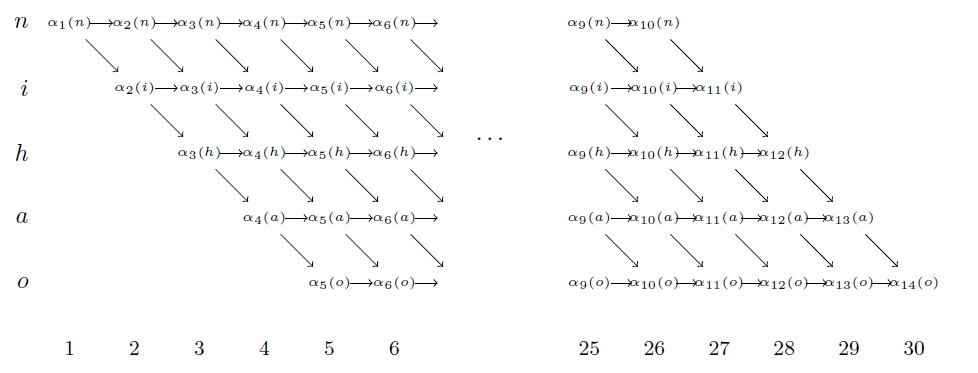即每个值都由上一个时刻的一个或者两个值得到，总计算量大约为$2.T.音素个数$$2.T.音素个数$。类似的，定义${\beta }_{t}\left(s\right)$$\beta_t(s)$， 递推公式为： ${\beta }_{14}\left(h\right)=\left({\beta }_{15}\left(h\right)+{\beta }_{15}\left(a\right)\right){y}_{h}^{14}$$\beta_{14}(h)=(\beta_{15}(h) + \beta_{15}(a))y^{14}_h$ 因此有： $\sum _{B\left(\pi \right)=z,{\pi }_{14}=h}\prod _{t=1}^{T}{y}_{{\pi }_{t}}^{t}=\left(前置项\right).{y}_{h}^{14}.$$\sum_{B(\pi)=z,\pi_{14}=h}\prod_{t=1}^T y^t_{\pi_t}= (前置项).y^{14}_h.$(后置项)$

=$\frac{{\alpha }_{14}\left(h\right)}{{y}_{h}^{14}}.{y}_{h}^{14}.\frac{{\beta }_{14}\left(h\right)}{{y}_{h}^{14}}$$\frac{\alpha_{14}(h)}{y^{14}_h}. y^{14}_h.\frac{\beta_{14}(h)}{y^{14}_h}$

=$\frac{{\alpha }_{14}\left(h\right){\beta }_{14}\left(h\right)}{{y}_{h}^{14}}$$\frac{\alpha_{14}(h)\beta_{14}(h)}{y^{14}_h}$

$\frac{\mathrm{\partial }p\left(z|y\right)}{\mathrm{\partial }{y}_{h}^{14}}$$\frac {\partial p(z|y)}{\partial y^{14}_h}$

= $\frac{\sum _{B\left(\pi \right)=z,{\pi }_{14}=h}\prod _{t=1}^{T}{y}_{{\pi }_{t}}^{t}}{\mathrm{\partial }{y}_{h}^{14}}$$\frac {\sum_{B(\pi)=z,\pi_{14}=h}\prod_{t=1}^T y^t_{\pi_t}}{\partial y^{14}_h}$

= $\frac{\frac{{\alpha }_{14}\left(h\right)}{{y}_{h}^{14}}.{y}_{h}^{14}.\frac{{\beta }_{14}\left(h\right)}{{y}_{h}^{14}}}{\mathrm{\partial }{y}_{h}^{14}}$$\frac {\frac{\alpha_{14}(h)}{y^{14}_h}. y^{14}_h.\frac{\beta_{14}(h)}{y^{14}_h}}{\partial y^{14}_h}$

=$\frac{{\alpha }_{14}\left(h\right){\beta }_{14}\left(h\right)}{{\left({y}_{h}^{14}\right)}^{2}}$$\frac{\alpha_{14}(h)\beta_{14}(h)}{{(y^{14}_h)}^2}$

©️2019 CSDN 皮肤主题: 编程工作室 设计师: CSDN官方博客